# Search ResultsCollection Resources(428)

View
Selected filters:
• FunctionsConditions of Use:
Remix and Share
Rating

The problem presents a context where a quadratic function arises. Careful analysis, ...

The problem presents a context where a quadratic function arises. Careful analysis, including graphing, of the function is closely related to the context. The student will gain valuable experience applying the quadratic formula and the exercise also gives a possible implementation of completing the square.

Subject:
Mathematics
Functions
Material Type:
Activity/Lab
Provider:
Illustrative Mathematics
Provider Set:
Illustrative Mathematics
Author:
Illustrative Mathematics
Date created
3-جمادي الأولى-1434Conditions of Use:
Remix and Share
Rating

The problem statement describes a changing algae population as reported by the ...

The problem statement describes a changing algae population as reported by the Maryland Department of Natural Resources. In part (a), students are expected to build an exponential function modeling algae concentration from the description given of the relationship between concentrations in cells/ml and days of rapid growth (F-LE.2).

Subject:
Mathematics
Functions
Material Type:
Activity/Lab
Provider:
Illustrative Mathematics
Provider Set:
Illustrative Mathematics
Author:
Illustrative Mathematics
Date created
3-جمادي الأولى-1434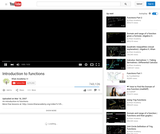Conditions of Use:
Remix and Share
Rating

This algebra lesson is an introduction to functions.

Subject:
Algebra
Functions
Material Type:
Lecture
Provider:
Provider Set:
Author:
Khan, Salman
Date created
3-ربيع الأول-1433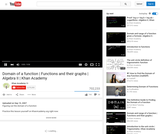Conditions of Use:
Remix and Share
Rating

An explanation of how to figure out the domain of a function.

Subject:
Algebra
Functions
Material Type:
Lecture
Provider:
Provider Set:
Author:
Khan, Salman
Date created
3-ربيع الأول-1433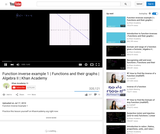Conditions of Use:
Remix and Share
Rating

A presentation of examples of variables varrying directly and inversely.

Subject:
Algebra
Functions
Material Type:
Lecture
Provider:
Provider Set:
Author:
Khan, Salman
Date created
3-ربيع الأول-1433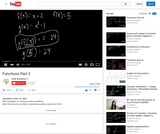Conditions of Use:
Remix and Share
Rating

This lesson demonstrates how to solve function problems.

Subject:
Algebra
Functions
Material Type:
Lecture
Provider:
Provider Set:
Author:
Khan, Salman
Date created
3-ربيع الأول-1433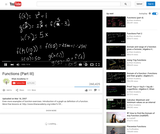Conditions of Use:
Remix and Share
Rating

This lesson concludes the demonstrations of function exercises and introduces the graph ...

This lesson concludes the demonstrations of function exercises and introduces the graph as a definition of a function.

Subject:
Algebra
Functions
Material Type:
Lecture
Provider:
Provider Set:
Author:
Khan, Salman
Date created
3-ربيع الأول-1433Conditions of Use:
Remix and Share
Rating

This is an example of a function problem that was submitted bya ...

This is an example of a function problem that was submitted bya YouTube viewer.

Subject:
Algebra
Functions
Material Type:
Lecture
Provider:
Provider Set:
Author:
Khan, Salman
Date created
3-ربيع الأول-1433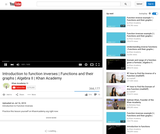Conditions of Use:
Remix and Share
Rating

An introduction to function inverses, and direct and inverse variation.

Subject:
Algebra
Functions
Material Type:
Lecture
Provider:
Provider Set:
Author:
Khan, Salman
Date created
3-ربيع الأول-1433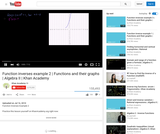Conditions of Use:
Remix and Share
Rating

An explanation of even and odd functions.

Subject:
Algebra
Functions
Material Type:
Lecture
Provider:
Provider Set:
Author:
Khan, Salman
Date created
3-ربيع الأول-1433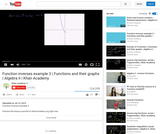Conditions of Use:
Remix and Share
Rating

This lesson gives a possible reason why even functions are called even, ...

This lesson gives a possible reason why even functions are called even, and odd functions are called odd.

Subject:
Algebra
Functions
Material Type:
Lecture
Provider:
Provider Set:
Author:
Khan, Salman
Date created
3-ربيع الأول-1433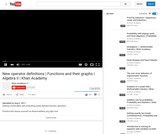Conditions of Use:
Remix and Share
Rating

This shows how a mathematician can define their own set of operators.

Subject:
Algebra
Functions
Material Type:
Lecture
Provider:
Provider Set:
Author:
Khan, Salman
Date created
3-ربيع الأول-1433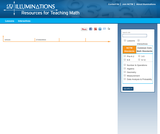Conditions of Use:
Rating

This two-lesson unit from Illuminations, exposes students to algebra, measurement, and data ...

This two-lesson unit from Illuminations, exposes students to algebra, measurement, and data analysis concepts and the major theme of analyzing change. In the first lesson, students measure the heights of classmates and older students and construct a table of height and age data to compare them. The second lesson's instructional goal is to understand how change in one variable, age, can relate to change in a second variable, height. Instructional plan, questions for the students, assessment options, extensions, and teacher reflections are given.

Subject:
Mathematics
Algebra
Functions
Material Type:
Interactive
Lesson Plan
Provider:
National Council of Teachers of Mathematics
Provider Set:
Illuminations
Author:
Elana Joram and Christina Hartman
Date created
12-محرم-1436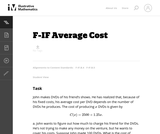Conditions of Use:
Remix and Share
Rating

In this real world problem students solve questions based on the relationship ...

In this real world problem students solve questions based on the relationship between production costs and price.

Subject:
Mathematics
Functions
Material Type:
Activity/Lab
Provider:
Illustrative Mathematics
Provider Set:
Illustrative Mathematics
Author:
Illustrative Mathematics
Date created
3-جمادي الأولى-1434Conditions of Use:
Remix and Share
Rating

This task provides a real world context for interpreting and solving exponential ...

This task provides a real world context for interpreting and solving exponential equations. There are two solutions provided for part (a). The first solution demonstrates how to deduce the conclusion by thinking in terms of the functions and their rates of change. The second approach illustrates a rigorous algebraic demonstration that the two populations can never be equal.

Subject:
Mathematics
Functions
Material Type:
Activity/Lab
Provider:
Illustrative Mathematics
Provider Set:
Illustrative Mathematics
Author:
Illustrative Mathematics
Date created
3-جمادي الأولى-1434Conditions of Use:
Rating

In this lesson, students model a bungee jump for a Barbie doll. ...

In this lesson, students model a bungee jump for a Barbie doll. They collect data in a table (number of rubber bands and jump distance), then create a scatterplot of the data, find its line of best fit, and write an equation of that line. Students go on to analyze the equation, noting its slope in relation to the data collected, and the meaning of the y-intercept. Since the distance to which the doll will fall is directly proportional to the number of rubber bands, the lesson provides a scenario for examining linear functions.

Subject:
Mathematics
Algebra
Functions
Numbers and Operations
Material Type:
Interactive
Lesson Plan
Provider:
National Council of Teachers of Mathematics
Provider Set:
Illuminations
Author:
Zordak, Samuel E.
Date created
20-شوال-1433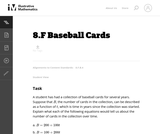Conditions of Use:
Remix and Share
Rating

This task could be put to good use in an instructional sequence ...

This task could be put to good use in an instructional sequence designed to develop knowledge related to students' understanding of linear functions in contexts. Though students could work independently on the task, collaboration with peers is more likely to result in the exploration of a range of interpretations.

Subject:
Mathematics
Functions
Material Type:
Activity/Lab
Provider:
Illustrative Mathematics
Provider Set:
Illustrative Mathematics
Author:
Illustrative Mathematics
Date created
1-جمادي الأولى-1434Conditions of Use:
Remix and Share
Rating

This task involves a fairly straightforward decaying exponential. Filling out the table ...

This task involves a fairly straightforward decaying exponential. Filling out the table and developing the general formula is complicated only by the need to work with a fraction that requires decisions about rounding and precision.

Subject:
Mathematics
Functions
Material Type:
Activity/Lab
Provider:
Illustrative Mathematics
Provider Set:
Illustrative Mathematics
Author:
Illustrative Mathematics
Date created
3-جمادي الأولى-1434Conditions of Use:
Remix and Share
Rating

This task describes two linear functions using two different representations. To draw ...

This task describes two linear functions using two different representations. To draw conclusions about the quantities, students have to find a common way of describing them. We have presented three solutions (1) Finding equations for both functions. (2) Using tables of values. (3) Using graphs.

Subject:
Mathematics
Functions
Material Type:
Activity/Lab
Provider:
Illustrative Mathematics
Provider Set:
Illustrative Mathematics
Author:
Illustrative Mathematics
Date created
1-جمادي الأولى-1434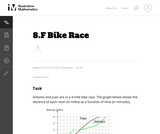Conditions of Use:
Remix and Share
Rating

The purpose of this task is for students to interpret two distance-time ...

The purpose of this task is for students to interpret two distance-time graphs in terms of the context of a bicycle race. There are two major mathematical aspects to this: interpreting what a particular point on the graph means in terms of the context, and understanding that the "steepness" of the graph tells us something about how fast the bicyclists are moving.

Subject:
Mathematics
Functions
Material Type:
Activity/Lab
Provider:
Illustrative Mathematics
Provider Set:
Illustrative Mathematics
Author:
Illustrative Mathematics
Date created
1-جمادي الأولى-1434